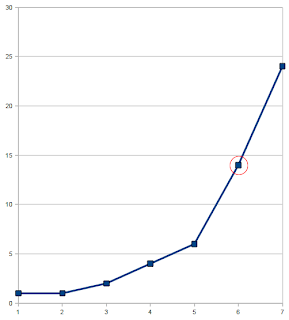## Tuesday, April 13, 2010

### Given a sequence of increasing values, find ...

The rationale of this post is hoping to find someone give me hints on a problem: Given a sequence of increasing values, find the first value which has a significant change in the sequence.

To visualize the problem: consider this sequence [1, 1, 2, 4, 6, 14, 24]
the "value" that has a significant change may be 14.

This can be plotted in a chart.The slope in between value 6 and 14 gives a significant change, obviously.

Indeed, this is a problem that i encountered when implementing the DBScan algorithm.

DBScan algorithm is a "density based" clustering algorithm. It takes two parameters - radius of a circle (eps), and the number of points in the circle which the circle can be considered as dense (minPts).

You may think DBScan as simple as... Taking any point, a "sphere" is formed with the radius (eps) center at that point. The circle may cover other points in a N-dimensions space. If the number of points covered by the "sphere" is larger than minPts, it is dense enough to form a cluster. And that cluster further expands with the points covered by that "sphere".

Finally ... points that are "closely" distanced will form a cluster and all points in the N-dimension space will form "many" cluster.

An important parameter in DBScan algorithm is the radius. From the wikipedia, the radius can be estimated by k-distance graph. First, let's define what is k-distance. Given a point, k-distance is distance between the k-th closest point of the given point. A k-distance graph is plotted by getting k-distance of all points in the N-dimension space, sorting them and plot it out as shown above. From the graph, it is simple to get the sharp change k-distance as the eps and using k as the minPts.

But, my concern is raised since it's not practical to plot 100 graph and read them all if i wanna try out k from 1 to 101. As consequence, i wanna have a algorithm to get the k-distance without looking into a visual graph.

One idea flow to my mind is to compare the slopes of neighbouring k-distance. If k-distance of pt-b is much larger than that of pt-a (the point just before pt-b), then k-distance of pt-b may probably be the selected sharp change k-distance. However, what is "much larger than" in this method? A parameter of floating point? Thus, i think this method is not that practical.

Another idea is to use the statistics - mean and standard deviation. Mean is the average while standard deviation is the square root of the variance. Variance is the average difference from the mean value of every point. So, standard deviation split the k-distance graph piece by piece fairly. If this is the case, I can compute the mean and make use of standard deviation to obtain a k-distance in several split away from the mean. By this method, the parameter is the number of split away from the mean value. An integer that can loop nicely :]

My implementation of this method is hosted on github #line23. Anyway, i believe there must be better method to obtain k-distance "automatically"... please comment :]